## Jack drops a stone from rest off of the top of a bridge that is 21.0 m above the ground. After the stone falls 7.0 m, Jill throws a second s

Question

Jack drops a stone from rest off of the top of a bridge that is 21.0 m above the ground. After the stone falls 7.0 m, Jill throws a second stone straight down. Both rocks hit the water at the exact same time. What was the initial velocity of Jill’s rock

in progress 0
2 months 2021-07-28T14:50:07+00:00 1 Answers 0 views 0

v = 19 m/s

Explanation:

• Since both rocks hit the water at the same time, and we know the total height of the bridge, we can get the time that it takes to the first rock to travel this height, due it is said that it was dropped, using the following kinematic equation: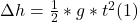• Replacing by the givens (Δh =21 m and g = 9.8 m/s2), and solving for t we get: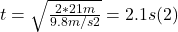• Now we need the time traveled by the first rock when it fell 7 m, that when the second rock was thrown, because the difference between the total time and this one will be the flight time for the second rock.
• We can use the same equation (1) replacing Δh by 7 m, and solving for t, in (2) as follows: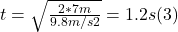• So, the total flight time for the second rock it was just the difference between (2) and (3):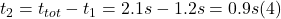• Since we know the total distance traveled, and the time of flight, we can use the same kinematic equation than in (1) but now taking into account the initial speed for the second rock, as follows:
•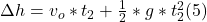• Since we know the value of Δh = 21 m and t₂ =0.9s, replacing in (5) and solving for v₀, we get: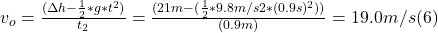• which was the initial velocity of Jill’s rock taking as positive the down ward direction.